# Polygon + triangle - math problems

#### Number of problems found: 81

• Goat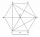The fenced flower bed has the shape of a regular hexagon. The tops are formed by fence posts. The fence around the flowerbed measures 60 m. A goat is tied to one of the pillars from the outside and grazes on the surrounding meadow (the goat should not ent
• Pentagonal pyramidThe height of a regular pentagonal pyramid is as long as the edge of the base, 20 cm. Calculate the volume and surface area of the pyramid.
• Hexaprism container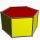Calculate the volume and surface in the shape of a regular hexagonal prism with a height of 1.4 m with a base edge of 3dm and a corresponding height of 2.6 dm.
• Pentagonal pyramidFind the volume and surface of a regular pentagonal pyramid with a base edge a = 12.8 cm and a height v = 32.1 cm.
• 9-gon pyramidCalculate the volume and the surface of a nine-sided pyramid, the base of which can be inscribed with a circle with radius ρ = 7.2 cm and whose side edge s = 10.9 cm.
• Hexagonal prism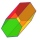Calculate the volume and surface of a regular hexagonal prism with the edge of the base a = 6 cm with the corresponding height v1 = 5.2cm and the height of the prism h = 1 dm.
• Find the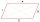Find the content of a regular 12 sided polygon if its side a = 12 cm.
• Inscribed and described circle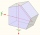Find the radii of a circle inscribed and circumscribed by a regular pentagon whose side measures 3 cm.
• Hexagonal pyramidCalculate the volume and surface area of a regular hexagonal pyramid with a base edge a = 30 m and a side edge b = 50 m.
• Hexagonal pyramidFind the volume of a regular hexagonal pyramid, the base edge of which is 12 cm long and the side edge 20 cm.
• Which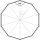Which of the following numbers most accurately area of a regular decagon with side s = 2 cm? (A) 9.51 cm2 (B) 20 cm2 (C) 30.78 cm2 (D) 31.84 cm2 (E) 32.90 cm2Calculate the content of a regular 15-sides polygon inscribed in a circle with radius r = 4. Express the result to two decimal places.
• DodecagonCalculate the size of the smaller of the angles determined by lines A1 A4 and A2 A10 in the regular dodecagon A1A2A3. .. A12. Express the result in degrees.
• Regular hexagonal prismCalculate the volume of a regular hexagonal prism whose body diagonals are 24cm and 25cm long.
• Interior angles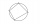In a quadrilateral ABCD, whose vertices lie on some circle, the angle at vertex A is 58 degrees and the angle at vertex B is 134 degrees. Calculate the sizes of the remaining interior angles.
• Triangular prismCalculate the surface of a regular triangular prism, the edges of the base are 6 cm long and the height of the prism is 15 cm.
• Five-gon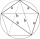Calculate the side a, the circumference and the area of the regular 5-angle if Rop = 6cm.
• AnnulusTwo concentric circles with radii 1 and 9 surround the annular circle. This ring is inscribed with n circles that do not overlap. Determine the highest possible value of n.
• Top of the towerThe top of the tower has the shape of a regular hexagonal pyramid. The base edge has a length of 1.2 m, the pyramid height is 1.6 m. How many square meters of sheet metal is needed to cover the top of the tower if 15% extra sheet metal is needed for joint
• Hexagonal pyramidCalculate the surface area of a regular hexagonal pyramid with a base inscribed in a circle with a radius of 8 cm and a height of 20 cm.

Do you have an exciting math question or word problem that you can't solve? Ask a question or post a math problem, and we can try to solve it.

We will send a solution to your e-mail address. Solved examples are also published here. Please enter the e-mail correctly and check whether you don't have a full mailbox.

Please do not submit problems from current active competitions such as Mathematical Olympiad, correspondence seminars etc...